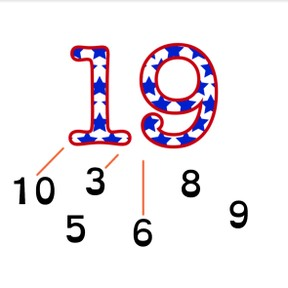No account needed.8,000 schools use Gynzy92,000 teachers use Gynzy1,600,000 students use Gynzy

## General

Students are able to determine which two or three addends are required to compose a given total of numbers to 20.

-1.OA.C

## Relevance

If students want to be able to calculate easily, it is important to be able to compose numbers to 20 with 2 or 3 addends. It helps to simplify the addition process and paves the way for subtraction. As an example, a class of 12 students can be made of different groups.

## Introduction

Show students an image on which they must count objects. Then they count dots on ladybugs. Ask students to count out loud to demonstrate their counting.

## Development

Discuss with students that you can compose numbers in different ways. Use the ladybugs as an example. Ask how many dots the ladybugs have together. Each pair has 12 dots. Explain that a number is made up of smaller numbers that total the larger number. Show different candles and ask which row has 14 candles. First find 14 with two kinds of candles, and then with three kinds of candles. By erasing the grey boxes, the answer is shown. Continue composing numbers of candles with students by dragging the candles to match the numbers given. First by using two numbers, and then with three.
Next, explain how students can determine which smaller numbers make up the larger number using the example with hamburgers. The students first take an addend from the number bank and count that many hamburgers. They count on to reach the total and then see if that second addend is also in the number bank. If the addend is in the number bank, then you have two addends to make the total. If the addend is not in the number bank, they must try another addend, or see if a third addend is available in the number bank. Discuss this with the example using flags. Finally have students practice determining the two addends of 16 and three addends of 18 with only the numbers being visible, without visual support. Students who are not ready to do this without support can use blocks to represent the numbers.

To check that students understand adding to 20 with 2 or 3 addends, you can ask the following questions:
- What do you do if you compose a number using 2 or 3 addends?
- Which addends can the total of 17 be made up of?
- Can the total 14 be composed of 4 addends?

## Guided practice

Students first practice adding a total with 2 addends with visual support. Then they practice adding a total with 3 addends with visual support. Finally students practice adding a total with 3 addends without visual support.

## Closing

Repeat the learning goal and ask what you do when you add a number with 2 or 3 addends. Which addends total 17? And can you compose 14 with 4 addends? Play a game in which students try to add to 20 with dice. Form groups of 3 or 4 students and give each group a 6, 10 and 12 sided dice. The students take turns throwing the dice and try to reach 20. The first student whose number adds to 20 gets a point.

## Teaching tips

Students who have difficulty with this can be supported by using blocks or other manipulatives. Practice with counting out the total number of blocks and then by making groups to show the different possibilities to add to the given total.

## Instruction materials

6, 10, and 12- sided dice, blocks

### The online teaching platform for interactive whiteboards and displays in schools

• Save time building lessons

• Manage the classroom more efficiently

• Increase student engagement